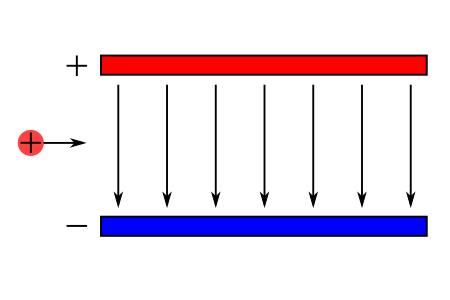# Show that charged particle entering a uniform electric field region follow a parabolic path.

## Question:

Show that charged particle entering a uniform electric field region follow a parabolic path.

## Coulomb Force and Electric Field:

The electric field E is the amount of electric force F per charge, {eq}E = F/q{/eq}. The direction of the electric field is radially outward from a positive charge and radially in toward a negative point charge.To solve the problem, let's refer to the figure above. A positive charge enters a uniform electric field that points downward. We can imagine a similar situation when a particle or object that is in the air and affected by the gravitational field. Hence, we can use kinematic equations to determine the motion of the charge along the horizontal and vertical directions. If the particle has an initial velocity of {eq}v_0{/eq}, then its horizontal velocity is

{eq}v = v_{0x}{/eq}.

It remains constant since the acceleration in this direction is zero. After some time t, the position of the particle is:

{eq}x = v_{0x}t{/eq}.

In the vertical direction, the charge experience a force (Coulomb) given by:

{eq}F = qE{/eq}

where q is the charge of the particle and E is the electric field. By Newton's Second Law of Motion, the acceleration in the downward direction is:

{eq}\begin{align*} F &= qE \\ \ \\ ma &= qE \\ \ \\ a &= \dfrac{qE}{m} \end{align*}{/eq}

Then, the velocity and position of the object in vertical direction are:

{eq}v_y = v_{0y}+ \left ( \dfrac{qE}{m} \right ) t{/eq}

{eq}y = v_{0y}t + \dfrac{1}{2}\left ( \dfrac{qE}{m} \right ) t^2{/eq}

Hence, the particle will follow a parabolic path when it enters a region of uniform electric field.